## Simulating diffraction efficiency of a volume holographic grating using Kogelnik’s method

Category: Product NewsThis blog post introduces the native volume hologram capabilities in OpticStudio that enable full simulation and analysis of holographic gratings in Sequential Mode considering their physical properties. The equivalent functionality is also demonstrated in Non-Sequential Mode by the use of DLLs. These analyses are important for designing systems such as head-up displays (HUD) and head-mounted displays (HMD) for use in virtual reality (VR) and augmented reality (AR). We’ll introduce the theory and parameters used in the model.

Sequential Mode volume holograms are available in all versions of OpticStudio however Diffraction Efficiency analysis is a Subscription only feature. The non-sequential DLL is a Premium Subscription feature.

Volume holograms are popular in many types of optical systems, where the ability of holograms to diffract rays to any desired angle, and their wavelength and angular selectivity allow the creation of lighter and more compact optical systems.

OpticStudio has supported the simulation of ideal holograms for a long time. However, to accurately account for the characteristics of volume holograms it is important to consider factors such as diffraction efficiency, material shrinkage, or index shift, in addition to the propagation direction of the diffracted rays. Considering diffraction efficiency allows advanced analyses like Image Simulation and comprehensive optimization.

Surface-Relief Grating vs. Volume Holographic Grating

Before introducing this model, let’s briefly explain the difference between a Surface-Relief Grating (SRG) and a Volume Holographic Grating (VHG). These two gratings are almost the same in terms of their role in the optical system but are quite different in terms of their fabrication and simulation.Figure 1. (a) Surface-Relief Grating (b) Volume Holographic Grating

• The VHG, shown in Figure 1 (b), is fabricated by exposing two or more beams on a light-sensitive emulsion film. The film is then chemically or thermally developed: that’s the grating. The surface of the grating is smooth, but the refractive index inside the grating varies sinusoidally. To model a VHG, algorithms such as efficient Kogelnik’s theory or robust Rigorous coupled-wave Analysis (RCWA) are required.

• The SRG, shown in Figure 1 (a), can be fabricated by several methods, such as e-beam writing, lithography, nanoimprinting, or diamond turning. Unlike the VHG, the SRG doesn’t have a spatially varying refractive index. Instead, the surface of the grating is made of periodic microstructures. To model an SRG, an algorithm like the Fourier Modal Method, also called RCWA, is required.

This post will present the tools for VHG.

See the Knowledgebase article, Simulating diffraction efficiency of the surface-relief grating using the RCWA method, for tools for SRG.

Two coupled-wave analyses

Let’s review the two coupled wave theories, which are mainly used in the Volume Holographic Grating model.

Consider a simple case that a hologram plane, with normal vector n, is illuminated by two plane waves of the same wavelength, propagated along with the directions of wave vectors 1 and k2. The plane waves are first refracted by Snell’s law when crossing the hologram and have new wave vectors k1 and k2 inside the hologram (Figure 2 (a)). The grating vector can then be defined by the following equation: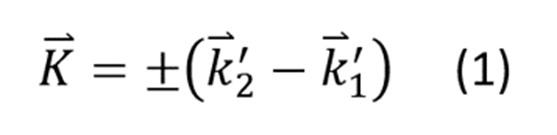After development, when the hologram is illuminated by a playback plane kp, the diffracted wave kd, can be determined by solving the equation:where the kp and kd are the wave vectors of playback and diffracted waves inside of the hologram emulsion (Figure 2 (b)). Note the grating vector K can be chosen from two reversed directions. The sign convention for equation (2) assumes the direction of K is chosen to satisfy K.kp >0.Figure 2. (a) Two construction beams refract into the hologram material (b) The playback ray refracts into the volume hologram

Now, we consider the fringes in the hologram are represented by sinusoidally varying refractive index n and α as in equation (3).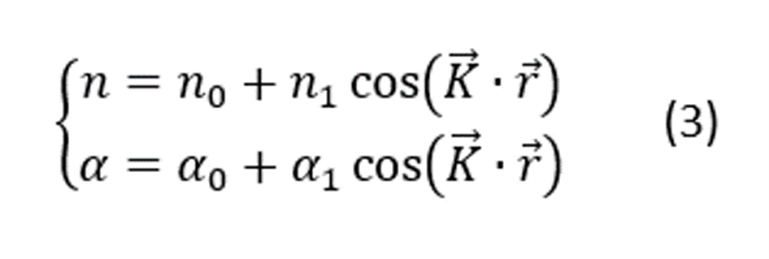Where n0 is the average index, n1 is the amplitude modulation of the refractive index and K is the grating vector.

The TE (transverse electric) polarized electric field of the diffracted and direct wave for transmission and reflection holograms can be calculated with the following 4 equations. Note, in this theory, it is assumed the energy only exchange between the incident, zero-order (direct wave), and first-order diffraction waves. To remove this limit, the theory of rigorous coupled-wave analysis is required.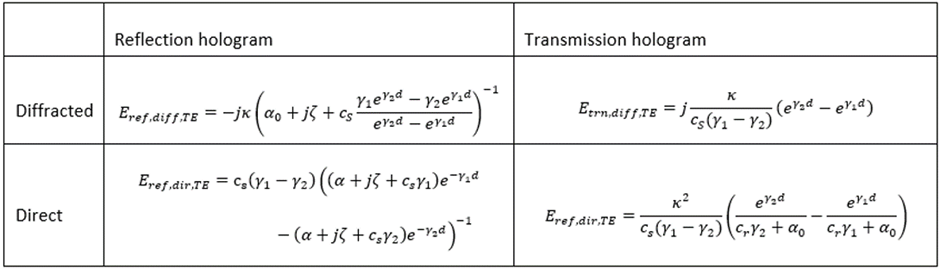were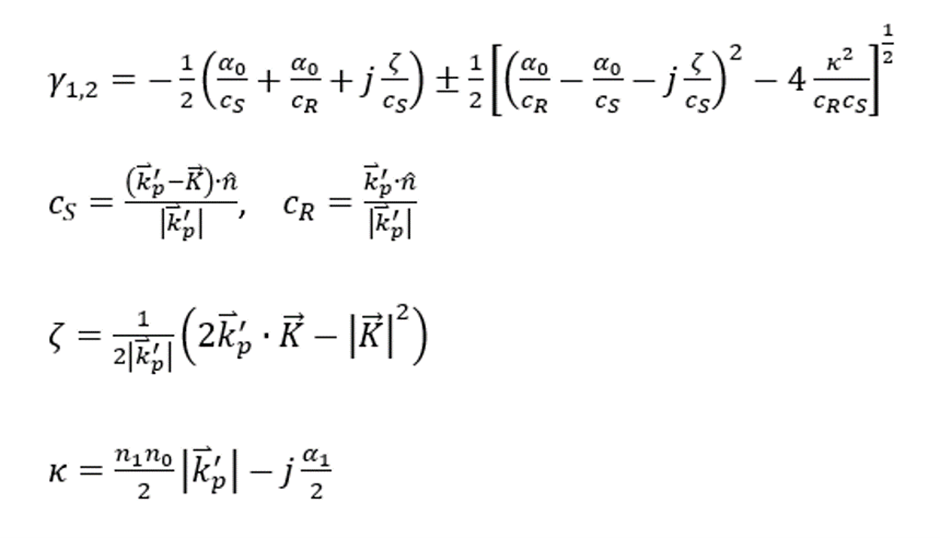For TM (Transverse Magnetic) polarization, we can simply replace the κ by κTM as follow and still use the previous equations.In the case of conical diffraction, where the incident ray is not perpendicular to the grating, the eigen polarization is defined as follows: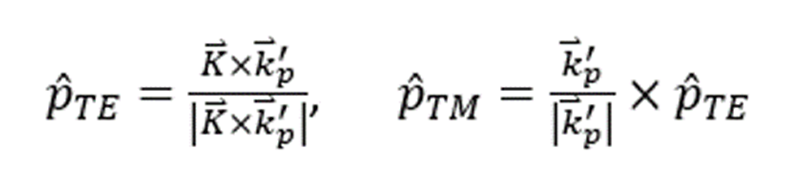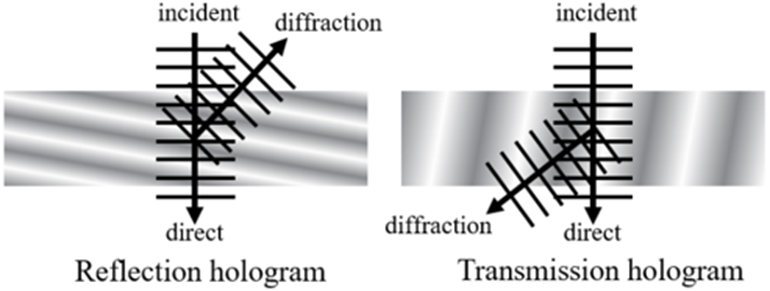Figure 3. In Kogelnik’s coupled wave theory, the hologram is thick enough that each incident ray either directly passes at the 0th order or is diffracted as the 1st order, for both reflection and transmission holograms.

Assumptions and limitations

Kogelnik’s Coupled Wave theory has the advantage over other theories that can predict accurately the response of the efficiency of the zero and first orders for volume phase gratings. However, this accuracy may decrease when either the thickness is low or when over-modulated patterns (high refractive index modulations) are recorded. It is therefore necessary to discuss the limits of applicability of Kogelnik’s theory for user’s reference.

• Index modulation: The index modulation cannot be too large compared to the average index. In other words, n1/n << 1. This is normally true for most practical cases. A rule of thumb is the ratio of n1/n should not be larger than 0.16 for reflection holograms and 0.06 for transmission holograms. 

• Thickness: The hologram is assumed to be thick. A rule of thumb is to ensure that:• Multi-order diffraction: This is the same limitation as to the thickness. For thick holograms, the energy of the input ray will be only transferred to either direct 0th or diffracted +1st order waves. For thin holograms, efficiency for other diffraction orders, such as -1 -2 +2 -3 +3 …, may not be zero.

• Multiplexing: There can only be one set of fringes existing in the hologram at once. Multiplexing of more than one fringe set is not allowed with Kogelnik’s method.

• Birefringent material: The hologram material is assumed to be isotropic. Birefringent material is not allowed.

Swelling/shrinkage

In this section, we will describe how the swelling and shrinkage of the hologram are considered.

When processing, the hologram emulsion may change its thickness. To consider the effect of thickness change, we first separate the grating vector into two components, K and K, where K is perpendicular and K is parallel to the surface normal. If thickness changes from t to t', the new grating vector can be calculated by modifying K as in equation (4).Figure 4. When the hologram emulsion shrinks, its thickness decreases from t to t’.

We’ve now covered the fundamentals of the volume hologram model. To learn more about how the theory is applied in OpticStudio, how to set up your sequential and non-sequential system, and to download example systems you can access the entirety of this Knowledgebase article here.